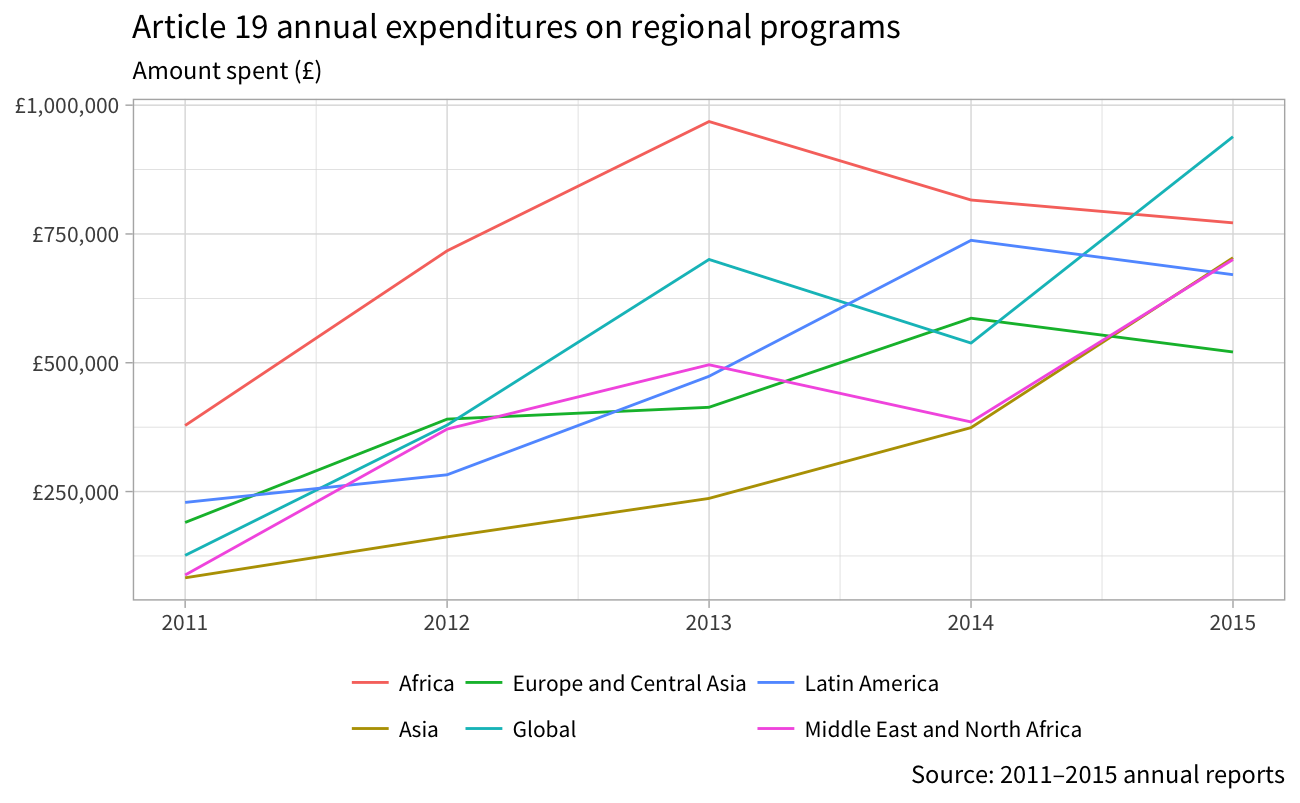Article 19 has generally increased its spending on regional programs evenly, with some notable exceptions. Prior to 2014, the bulk of its spending was directed to programs in Africa, wih a peak in 2013 of nearly £1,000,000. In the past couple years, however, African spending has been reduced, while global programs have increased, possibly as a replacement (though not entirely, since global programs were the second most funded category in 2013)

``````library(tidyverse)
library(ggstance)
a19 <- read_csv("~/Desktop/a19.csv") %>%
filter(!(Category %in% c("Governance", "Fundraising", "Law and policy"))) %>%
group_by(Year, Category) %>%
summarise(Amount = sum(Amount))``````
``````Parsed with column specification:
cols(
Year = col_integer(),
Subcategory = col_character(),
Category = col_character(),
Amount = col_integer()
)``````
``````a19.plot.amount <- ggplot(a19, aes(x=Year, y=Amount, colour=Category)) +
geom_line() +
guides(colour=guide_legend(title=NULL)) +
labs(x=NULL, y=NULL,
title="Article 19 annual expenditures on regional programs",
subtitle="Amount spent (£)",
caption="Source: 2011–2015 annual reports") +
scale_y_continuous(labels=scales::dollar_format(prefix="£")) +
theme_light(base_family="Source Sans Pro") +
theme(legend.position="bottom")
a19.plot.amount``````Looking at the percent of money spent on regional programs highlights this shift even better. Africa had been receiving roughly 30% of all funding until 2014, when Asia became more prominent. By 2015, all regions get between 12 and 22 percent.

``````a19.percent <- a19 %>%
group_by(Year) %>%
mutate(total = sum(Amount),
percent = Amount / total)
a19.plot.percent <- ggplot(a19.percent, aes(x=Year, y=percent, colour=Category)) +
geom_line() +
guides(colour=guide_legend(title=NULL)) +
labs(x=NULL, y=NULL,
title="Article 19 annual expenditures on regional programs",
subtitle="% of total spending",
caption="Source: 2011–2015 annual reports") +
scale_y_continuous(labels=scales::percent) +
theme_light(base_family="Source Sans Pro") +
theme(legend.position="bottom")
a19.plot.percent``````